# Civil Engineering - Structural Design Specifications

### Exercise :: Structural Design Specifications - Section 3

41.

The total area of side face reinforcement, in case of a deep beam should not be less than

 A. 0.1% of web area B. 0.2% of web area C. 0.3% of web area D. 0.4% of web area.

Explanation:

No answer description available for this question. Let us discuss.

42.

As per IS : 1982, the permissible axial tension in rolled I-beams and channels, is

 A. 1500 kg/cm2 B. 1800 kg/cm2 C. 1400 kg/cm2 D. 1420 kg/cm2

Explanation:

No answer description available for this question. Let us discuss.

43.

The thickness of flat single lacing is, taken (where l is effective length of the bar).

 A.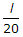B.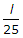C.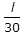D.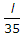E.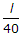Explanation:

No answer description available for this question. Let us discuss.

44.

The average 28 days compressive strength of at least three 15 cm concrete cubes prepared with available water as compared to the average strength of three similer concrete cubes prepared with distilled water, should be at least

 A. 70% B. 80% C. 90% D. 95%

Explanation:

No answer description available for this question. Let us discuss.

45.

In the formula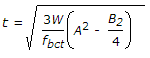A. A and B are the dimensions of the column B. A and B are the dimensions of the base plate C. A is the greater projection of the plate beyond the column D. B is the lesser projection of the plate beyond the column E. Both (c) and (d) of above.